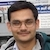# NAEST 2018 Screening Test: Force on the GuavaBy

A guava is kept on a hard surface. It is cut into two equal halves by a knife moving in a vertical plane. What is the resultant force on the guava?

Question: Assume that the knife divides the guava equally in two parts. During the cutting operation (before the guava separates), the resultant force on the guava is

1. Zero
2. In the direction of the movement of knife
3. Opposite to the direction of motion of the knife
4. Perpendicular to the direction of motion of the knife.

Solution: The knife cuts guava into two symmetrical parts. The centre of mass of the guava remains fixed during the cutting operation. Thus, the acceleration of the centre of mass is zero. Hence, By Newton's second law, the resultant force on the guava is zero during the cutting operation.

The downward force applied by the knife on the guava is balanced by the reaction from the ground. The horizontal forces applied to the two parts are equal and opposite due to symmetrical cutting.

The questions in textbooks usually ask for a body's acceleration for given forces on it. In real life, a machine designer has to solve a reverse problem. He needs to find forces that can generate desired acceleration. The question on guava cutting represents a real problem and not a hypothetical textbook problem.

See an explanation of this question by Dr HC Verma.